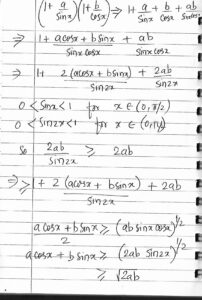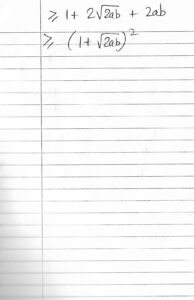Selected Sample Problems and Solutions

Problem 1: If a, b, c are three positive real numbers  such that  , then find the maximum value of the expression ?

Solution:  Use   inequality on       to get the answer as 27 .

Detailed solution: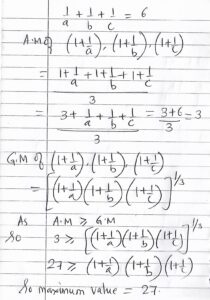Problem 2:  In problem 1, find the minimum value of       ?

Solution: Use     inequality on     to get the answer as 12 . [QM and AM stand for quadratic and arithmetic means respectively].

Detailed solution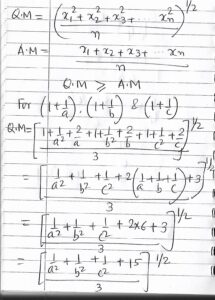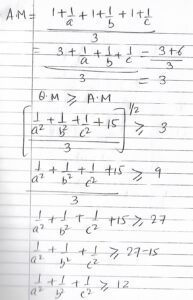Problem 3:  Find the value of the limit

Solution:   . Detailed solutionProblem 4:  Find the value of the limit

Solution: 1. Detailed solution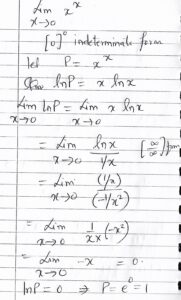Problem 5: If is a real number and   , find the value of   ?

Solution:  1/2 . Detailed solution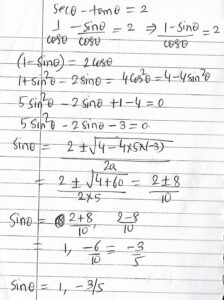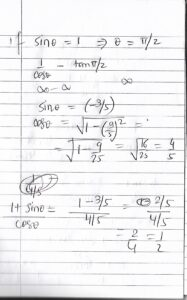Problem 6:  Find the minimum value of the  below expression for all real values of     ?

Solution:

Problem 7 : For positive real numbers a, b, x  such that   and    show that

Solution: